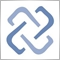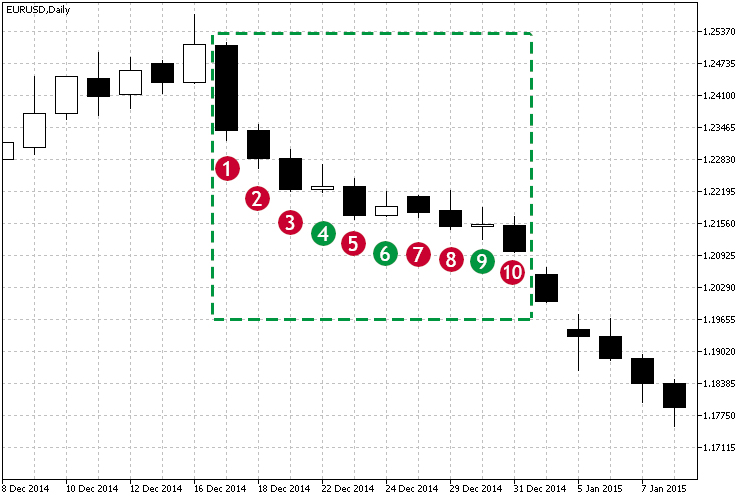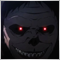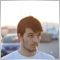# Discussion of article "Practical application of correlations in trading"Moderator
235152

New article Practical application of correlations in trading has been published:

In this article, we will analyze the concept of correlation between variables, as well as methods for the calculation of correlation coefficients and their practical use in trading. Correlation is a statistical relationship between two or more random variables (or quantities which can be considered random with some acceptable degree of accuracy). Changes in one ore more variables lead to systematic changes of other related variables.

Correlation is a statistical relationship between two or more random variables (or quantities which can be considered random with some acceptable degree of accuracy). Changes in one ore more variables lead to systematic changes of other related variables. The mathematical measure of the correlation of two random variables is the correlation coefficient. If a change in one random variable does not lead to a regular change in the other random variable but leads to a change in another statistical characteristic of this random variable, such a relation is not considered correlation, although it is statistical.

The correlation coefficient values can vary from -1 to +1. The closer the correlation value to 1, the higher the interdependence between the variables studied. And if the value tends to 1, correlation is considered positive. If the value tends to -1, correlation is negative. During positive correlation, an increase in one of the variables leads to an increase in the second one. In case with the negative correlation, an increase in one value leads to a decrease in the second one.

In other worlds, correlations helps identify the dependence of one variable on the second one based on the available data. How can correlation help in financial market trading?

Let us view Fig.1 and the marked downtrend zone.Author: Alexander Fedosov295

Very interesting. Thank you.2308

Good job, but only relevant results on EURUSD as it was coded.